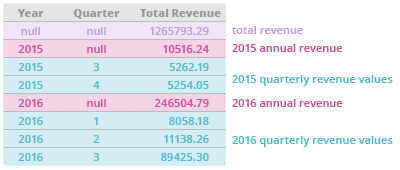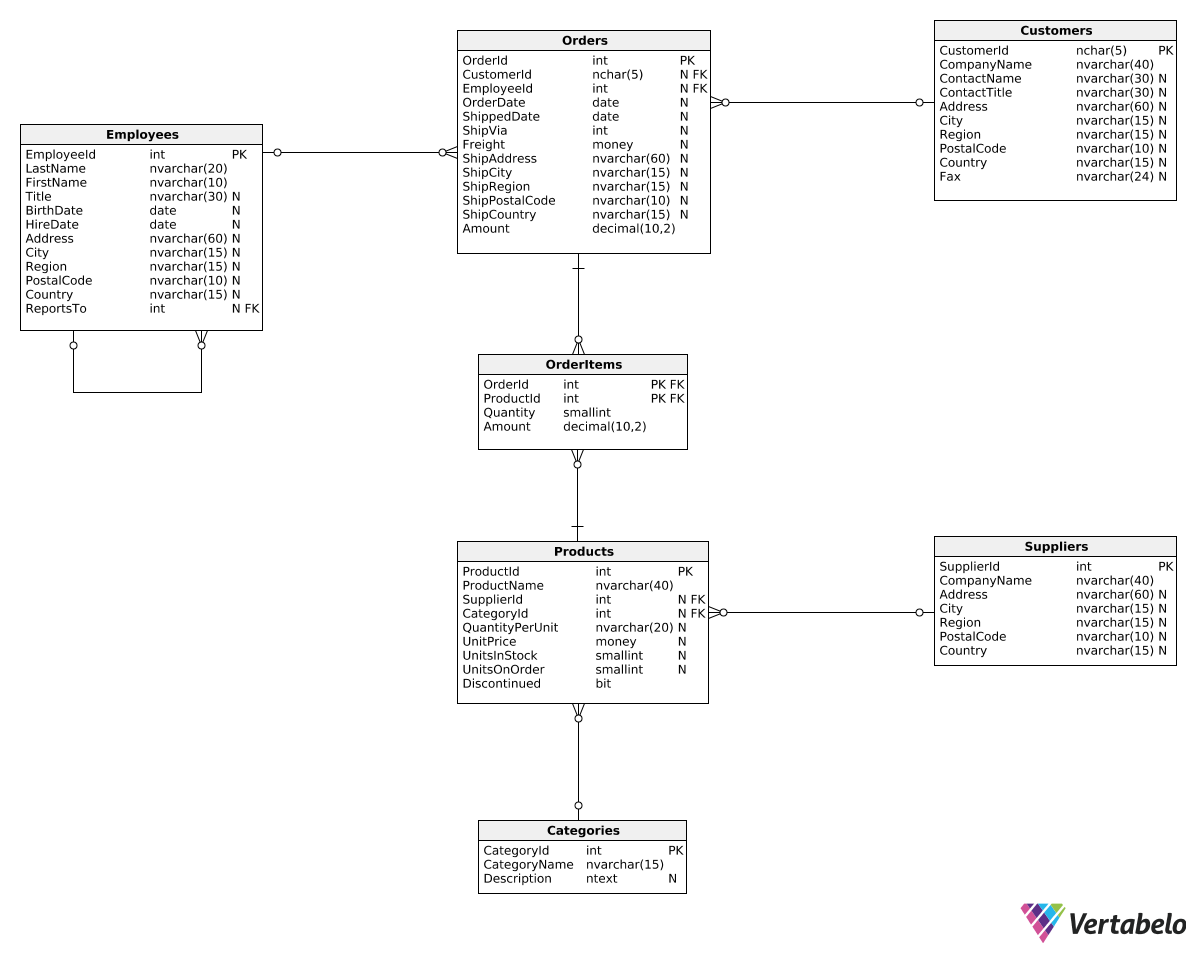Deals Of The Week - hours only!Up to 80% off on all courses and bundles.-Close
Introduction
Total revenue for multiple periods
Calculating deltas
Creating "revenue in quarters" reports
Summary

## Instruction

Very well done! In the previous explanation, we calculated the quarterly revenue in each year. Now, let's say we want a report containing:

1. quarterly revenue values.
2. annual revenue values.
3. the grand total revenue value.

Here's an example of the result we'd like to achieve:Sound complicated? Here's a query to handle it:

SELECT
DATEPART(Year, OrderDate) AS RevenueYear,
DATEPART(Quarter, OrderDate) AS RevenueQuarter,
SUM(Amount) AS TotalRevenue
FROM Orders
GROUP BY ROLLUP(
DATEPART(Year, OrderDate),
DATEPART(Quarter, OrderDate)
)
ORDER BY
DATEPART(Year, OrderDate),
DATEPART(Quarter, OrderDate);


We introduced a small change in the GROUP BY clause. Instead of:

GROUP BY
DATEPART(Year, OrderDate),
DATEPART(Quarter, OrderDate)

we used:

GROUP BY ROLLUP(
DATEPART(Year, OrderDate),
DATEPART(Quarter, OrderDate)
)

Let's run the query and see what happens.

## Exercise

Run the template query.

As you can see, we've got rows showing three aggregation levels: the grand total revenue, annual revenues, and the quarterly revenues for each year.

In other words, now you can see:

1. the grand total revenue – the total sum for all years (RevenueYear and RevenueQuarter being NULL).
2. the annual revenues – the sums for each year (RevenueQuarter being NULL).
3. the sums for each year and quarter (being the results of the query from the pevious exercise).

### Stuck? Here's a hint!